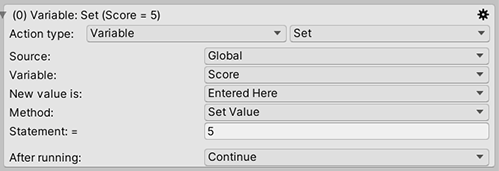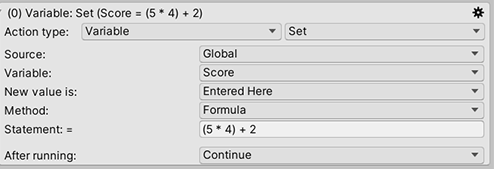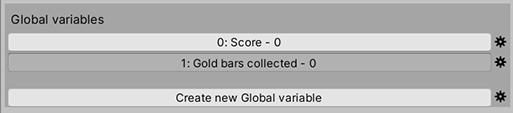# Setting variables using mathematical formulas

Adventure Creator supports Global Variables in the form of strings, integers, booleans and floats. Variables are declared in the Variables Manager, and their values can be changed at runtime using the Variable: Set Action. For example, this Action is being used to set the value of the Score integer to 5:When manipulating integers and floats, we have the option of entering a mathematical formula, rather than just a single value, by changing the Method to Formula. Note, however, that this feature is not available in Android games.

As an example, the following Action will give the Score integer a value of 22:This feature is at it's most valuable when we use the values of other Variables in our formula. For example, we may wish our score to be larger based on how many gold bars we've collected.

Let's assume that the number of gold bars we've collected is stored in another integer Variable:In the Gold bars collected properties section, we've set the Initial value to 9 (for demonstration purposes). We can also see a field for Replacement token. This is the text we must enter into our formula box, which will be substituted internally for the Variable's value.Therefore, the following formula will set the Score integer to be three times the value of Gold bars collected, or 27.